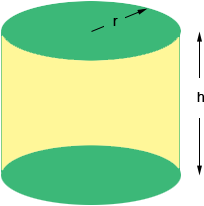I have a Cylinder which is 34" x 72" how many gallons will it hold? Doug If a cylinder has base radius r inches and height h inches then the volume is V =r2 h cubic inchesWhen you say you have a cylinder that is 34 "72" I assume you mean that the diameter of the base is 34" and the height is 72". In this case the radius of the base is 17 " and hence the volume is V =17272 = 65370.3 cubic inches. There are 231 cubic inches in an american gallon and hence the volume of your cylinder is  65370.3/231 = 283.0 gallons Penny